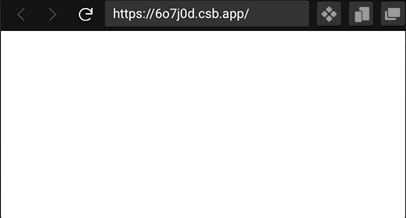# Creating an animated typing effect in ReactThe idea behind this is that we render text from the state and keep updating state variable one character at a time with a bit of delay.

Each time state is updated our component will re-render showing text "typed" one character after another.

So first thing in our React app we will create a component. Let's name it `TextTyper`. We will need to have `useState` and `useEffect` hooks in it, so we can import them right away:

``````import React, { useState, useEffect } from "react";

const TextTyper=() => {
const [typedText, setTypedText] = useState("");

return <span>{typedText}</span>
}

export default TextTyper
``````

In state we have declared a variable `typedText` which is rendered inside `span` HTML element. This would be the variable which we are going to update.

Let's say we want to print "Banana". First we will render empty string, then we will add "B" to `typedText` and see it in the page, then we will update it to "Ba", and so on...

Let's create a function `typingRender`. It will take 3 arguments: the whole phrase to type, the updater method which will be our `setTypedText` and an interval value to tell the function the delay between rendering each character.

In this function we will use variable `localTypingIndex` to go through the indexes of characters in the incoming string and take them one by one. We will also declare a variable `localTyping` to mirror what should be in state and add letters to it to put to state:

``````import React, { useState, useEffect } from "react";

const TextTyper=() => {
const [typedText, setTypedText] = useState("");

const typingRender = (text, updater, interval) => {
// local counter used to go through indexes of the phrase
let localTypingIndex = 0;
// local string to add characters to and put to state
let localTyping = "";
if (text) {
// if we have a phrase to type we will start the interval
let printer = setInterval(() => {
// if our local index counter is less than the length of the phrase we keep going
if (localTypingIndex < text.length) {
// we set to state our local string with the phrase
updater((localTyping += text[localTypingIndex]));
// and increase the local index
localTypingIndex += 1;
} else {
// once we reached the end of the phrase we reset local index
localTypingIndex = 0;
// clear local string with phrase
localTyping = "";
// clear the interval to stop
clearInterval(printer);
}
}, interval);
}
};

return <span>{typedText}</span>
}

export default TextTyper
``````

Now we have our typing function it's time to execute it. We want this to happen once when component is initially rendered so we will use `useEffect` with empty array of dependencies:

``````const TextTyper=() => {
const [typedText, setTypedText] = useState("");
// declare the variable to hold the phrase
const text = 'This will be the phrase printed one by one character'
// declare a variable with the interval of 100 milliseconds
const interval = 100
const typingRender = (text, updater, interval) => {
let localTypingIndex = 0;
let localTyping = "";
if (text) {
let printer = setInterval(() => {
if (localTypingIndex < text.length) {
updater((localTyping += text[localTypingIndex]));
localTypingIndex += 1;
} else {
localTypingIndex = 0;
localTyping = "";
clearInterval(printer);
}
}, interval);
}
};
// run this effect on first render
useEffect(() => {
// call the function passing a phrase, setter method for state and interval var
typingRender(text, setTypedText, interval);
}, [text, interval]);

return <span>{typedText}</span>
}

export default TextTyper
``````

Now the component will work perfectly fine but we can make it more flexible, so all the data for it to work will come via props and we will even set the variable to control inside which HTML element we want to render our phrase:

``````import React, { useState, useEffect } from "react";

const TextTyper=({
// now the phrase, interval and HTML element desired will come via props and we have some default values here
text = "",
interval = 100,
Markup = "span"
}) => {
const [typedText, setTypedText] = useState("");

const typingRender = (text, updater, interval) => {
let localTypingIndex = 0;
let localTyping = "";
if (text) {
let printer = setInterval(() => {
if (localTypingIndex < text.length) {
updater((localTyping += text[localTypingIndex]));
localTypingIndex += 1;
} else {
localTypingIndex = 0;
localTyping = "";
clearInterval(printer);
}
}, interval);
}
};
useEffect(() => {
typingRender(text, setTypedText, interval);
}, [text, interval]);

return <Markup>{typedText}</Markup>
}

export default TextTyper
``````

So now if we want to use this we can import the component and render it with passing some props:

``````// importing our component in App.js
import TextTyper from './TextTyper'

function App() {
const textToRender = 'This will be the phrase printed one by one character'
return (
<div className="App">
{/* rendering it passing */}
<TextTyper text={textToRender} interval={10} Markup={"code"} />
</div>
);
}

export default App;
``````

Here is a link to the working code sandbox.

You can also install and import this component with `npm` or `yarn`

Back to posts